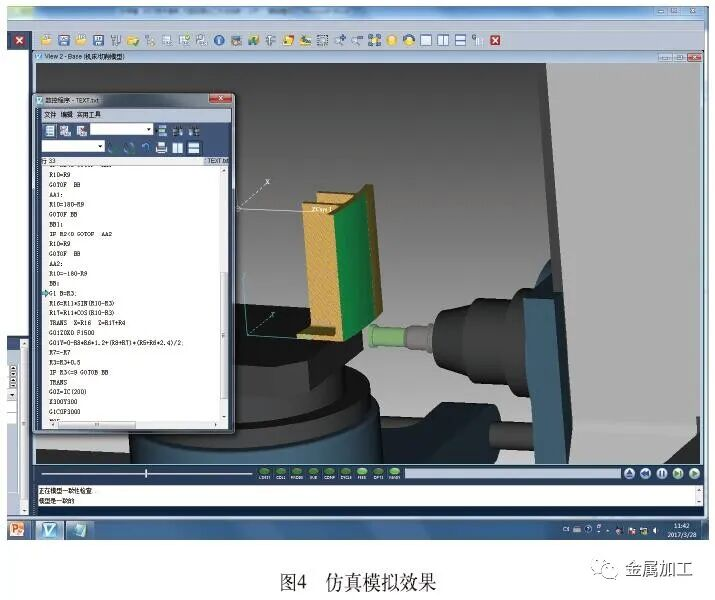## 拥有核心技术的雕铣机厂家

13年来 • 我们不断让数控机床变得更好

4009-630-833

## 资讯中心

http://www.xwhcnc.com/

# 大弧面四轴如何加工

1.加工分析

2. 技术方案

（1）数学模型分析  图2中三张图片分别为工件装夹初始位置、加工初始位置和加工终点位置三种状态下的示意图，其中O点为工作台的中心位置，A点为加工的起始位置，B点为终止位置，C点为圆弧的初始位置

（2）宏程序编制 程序逻辑控制图如图3所示。先对各参数赋值，然后依据弧面中心的坐标数值，对其所在的象限进行自动判别。对象限判别的目的是为了计算圆弧中心和工作台中心（零点位置）连线与Z轴正向之间的夹角。最后，进入加工阶段，开始按宏程序进行往复加工。

G54；设定坐标系

G64；启动连续切削模式

SOFT；圆滑增减速打开

FFWON；前馈控制打开

T1D1；调用φ50端面铣刀，选择1号刀具，调用1号长度补偿；用Vericut仿真时更改为T1M6

G0 X300Y60；初始定位Z500；快速运动到安全位置S1300M03；

R1=100；初始装夹位置R745圆弧中心X坐标

R2=-515；初始装夹位置 R745圆弧中心Z坐标

R3=-9；加工初始状态角度初值

R4=745；工件圆弧半径

R5=280；弧面长度

R6=25；刀具半径

R7=-1；方向控制变量，初始向下，设置为-1

R8=R7；圆弧中心位置判断及初始角度计算

R11=SQRT(R1*R1+R2*R2)；圆弧中心相对工作台中心旋转半径

R 9 = A S I N ( R 1 / S Q R T ( R 1 * R 1 + R2*R2))；

IF  R1<0 GOTOF BB1；满足条件跳转，否则进行一、二象限角度判别

IF R2<0 GOTOF  AA1；

R10=R9；第一象限角度判别计算

GOTOF  BB；完成后跳出判别区域 AA1：

R10=180-R9；第二象限，角度判别计算

GOTOF BB；完成后跳出判别区域BB1：

IF R2<0 GOTOF AA2；第三、四象限初始角度判别

R10=R9

GOTOF  BB

AA2:

R10=-180-R9；判别结束

BB：

G1 B=R3；

R16=R11*SIN(R10-R3)；圆弧中心X坐标值

R17= R11*COS(R10-R3)；圆弧中心Z坐标值

TRANS  X=R16  Z=R17+R4；坐标偏移

G01Z0F2000；Z轴定位

G01X0F1500；X轴定位

G01Y=0-8*R6*1.2+(R8+R7)*(R5+ R6*2.4)/2；数字1.2为单边延伸系数。2.4为两边总延伸系数。

R7=-R7

R3=R3+0.5；度数递增

IF R3<=9 GOTOB BB；满足条件跳转

TRANS；坐标偏移取消

G0Z=IC(200)；Z向退刀

X300Y300；移动到安全位置

G1C0F3000；工作台旋转至初始位置

M05；主轴停止

M09；冷却液关闭

M30；程序结束，系统复位

①在ZOX平面内进行角度计算，Z为横轴，X为纵轴。②注意工作台旋转时，顺时针为正，而坐标系逆时针旋转 为 正 。③ 圆 弧 初 始 位 置 坐 标值，通过对刀确定。④工作台的中心坐标，建议使用基准块进行校准，这样有利于减少零件的加工误差。3. 结语

## 最新资讯

﻿

•官方二维码

 鑫腾辉客服